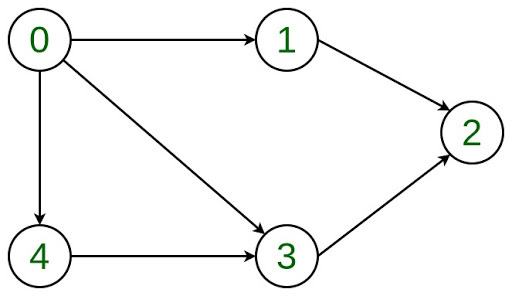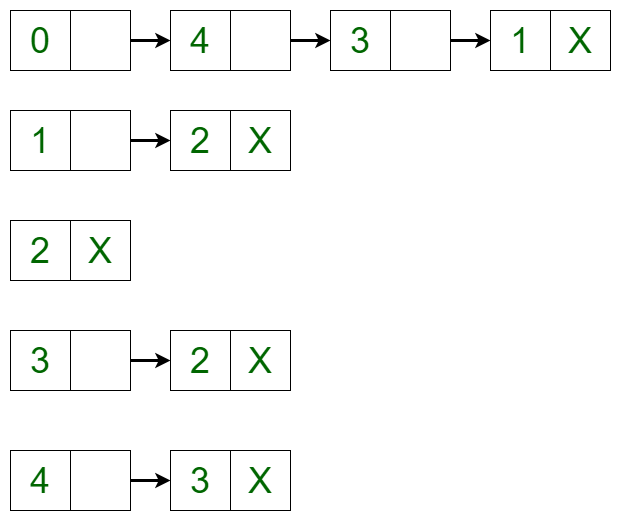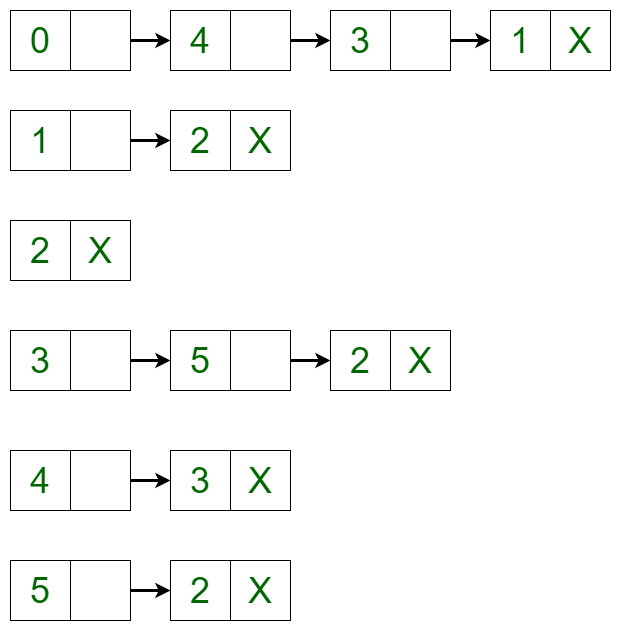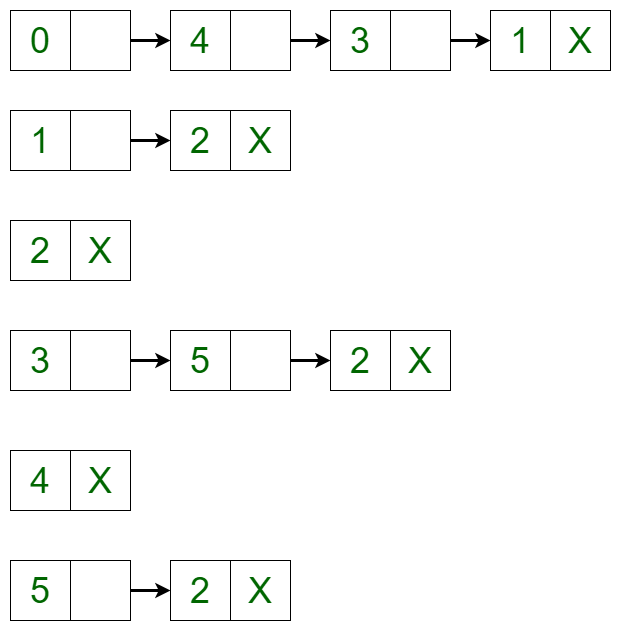Prerequisites: Linked List, Graph Data Structure

Let the Directed Graph be:The graph can be represented in the Adjacency List representation as:It is a Linked List representation where the head of the linked list is a vertex in the graph and all the connected nodes are the vertices to which the first vertex is connected. For example, from the graph, it is clear that vertex 0 is connected to vertex 4, 3 and 1. The same is representated in the adjacency list(or Linked List) representation.

## Recommended: Please try your approach on {IDE} first, before moving on to the solution.

Approach:

The idea is to represent the graph as a list of linked lists where the head of the linked list is the vertex and all the connected linked lists are the vertices to which it is connected.

• Adding a Vertex in the Graph: To add a vertex in the graph, the adjacency list can be iterated to the place where the insertion is required and the new node can be created using linked list implementation. For example, if 5 needs to be added between vertex 2 and vertex 3 such that vertex 3 points to vertex 5 and vertex 5 points to vertex 2, then a new edge is created between vertex 5 and vertex 3 and a new edge is created from vertex 5 and vertex 2. After adding the vertex, the adjacency list changes to:• Removing a Vertex in the Graph: To delete a vertex in the graph, iterate through the list of each vertex if an edge is present or not. If the edge is present, then delete the vertex in the same way as delete is performed in a linked list. For example, the adjacency list translates to the below list if vertex 4 is deleted from the list:Below is the implementation of the above approach:

 `# Python implementation of the above approach ` `# Implementing Linked List representation ` `class` `AdjNode(``object``): ` `    ``def` `__init__(``self``, data): ` `        ``self``.vertex ``=` `data ` `        ``self``.``next` `=` `None` ` `  `# Adjacency List representation ` `class` `AdjList(``object``): ` ` `  `    ``def` `__init__(``self``, vertices): ` `        ``self``.v ``=` `vertices ` `        ``self``.graph ``=``[``None``]``*``self``.v ` ` `  `# Function to add an edge from a source vertex  ` `# to a destination vertex ` `    ``def` `addedge(``self``, source, destination): ` `        ``node ``=` `AdjNode(destination) ` `        ``node.``next` `=` `self``.graph ` `        ``self``.graph``=` `node ` ` `  `# Function to call the above function. ` `    ``def` `addvertex(``self``, vk, source, destination): ` `        ``graph.addedge(source, vk)  ` `        ``graph.addedge(vk, destination) ` ` `  `# Function to print the graph ` `    ``def` `print_graph(``self``): ` `        ``for` `i ``in` `range``(``self``.v): ` `            ``print``(i, end ``=``"") ` `            ``temp ``=` `self``.graph[i] ` `            ``while` `temp: ` `               ``print``(``"->"``, temp.vertex, end ``=``"") ` `               ``temp ``=` `temp.``next` `            ``print``(``"\n"``) ` ` `  `# Function to delete a vertex ` `    ``def` `delvertex(``self``, k): ` ` `  `# Iterating through all the vertices of the graph ` `        ``for` `i ``in` `range``(``self``.v): ` `            ``temp ``=` `self``.graph[i] ` `            ``if` `i ``=``=` `k: ` `                ``while` `temp: ` `                    ``self``.graph[i]``=` `temp.``next` `                    ``temp ``=` `self``.graph[i] ` `                     `  `            ``# Delete the vertex  ` `            ``# using linked list concept         ` `            ``if` `temp: ` `                ``if` `temp.vertex ``=``=` `k: ` `                    ``self``.graph[i]``=` `temp.``next` `                    ``temp ``=` `None` `            ``while` `temp: ` `                ``if` `temp.vertex ``=``=` `k: ` `                    ``break` `                ``prev ``=` `temp ` `                ``temp ``=` `temp.``next` ` `  `            ``if` `temp ``=``=` `None``: ` `                ``continue` ` `  `            ``prev.``next` `=` `temp.``next` `            ``temp ``=` `None` `     `  ` `  `# Driver code ` `if` `__name__ ``=``=` `"__main__"``: ` ` `  `    ``V ``=` `6` `    ``graph ``=` `AdjList(V)  ` `    ``graph.addedge(``0``, ``1``) ` `    ``graph.addedge(``0``, ``3``) ` `    ``graph.addedge(``0``, ``4``) ` `    ``graph.addedge(``1``, ``2``) ` `    ``graph.addedge(``3``, ``2``) ` `    ``graph.addedge(``4``, ``3``) ` ` `  `print``(``"Initial adjacency list"``) ` `graph.print_graph()  ` ` `  `# Add vertex ` `graph.addvertex(``5``, ``3``, ``2``) ` `print``(``"Adjacency list after adding vertex"``) ` `graph.print_graph()  ` ` `  `# Delete vertex ` `graph.delvertex(``4``) ` `print``(``"Adjacency list after deleting vertex"``) ` `graph.print_graph() `

Output:

```Initial adjacency list
0-> 4-> 3-> 1

1-> 2

2

3-> 2

4-> 3

5

0-> 4-> 3-> 1

1-> 2

2

3-> 5-> 2

4-> 3

5-> 2

0-> 3-> 1

1-> 2

2

3-> 5-> 2

4

5-> 2
```

GeeksforGeeks has prepared a complete interview preparation course with premium videos, theory, practice problems, TA support and many more features. Please refer Placement 100 for details

My Personal Notes arrow_drop_upCheck out this Author's contributed articles.

If you like GeeksforGeeks and would like to contribute, you can also write an article using contribute.geeksforgeeks.org or mail your article to contribute@geeksforgeeks.org. See your article appearing on the GeeksforGeeks main page and help other Geeks.

Please Improve this article if you find anything incorrect by clicking on the "Improve Article" button below.